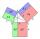# Pythagorean theorem - math word problems

1. Short cutImagine that you are going to the friend. That path has a length 120 meters. Then turn doprava and go another 630 meters and you are at a friend's. The question is how much the journey will be shorter if you go direct across the field?
2. IS trapezoidCalculate the length of diagonal u and height v of isosceles trapezoid ABCD, whose bases have lengths a = |AB| = 37 cm, c = |CD| = 29 cm and legs b = d = |BC| = |AD| = 28 cm.
3. Spherical capFrom the sphere of radius 13 was truncated spherical cap. Its height is 6. What part of the volume is spherical cap from whole sphere?
4. MediansCalculate the sides of a right triangle if the length of the medians to the legs are ta = 21 cm and tb=12 cm.
5. RhombusIt is given a rhombus of side length a = 29 cm. Touch points of inscribed circle divided his sides into sections a1 = 14 cm and a2 = 15 cm. Calculate the radius r of the circle and the length of the diagonals of the rhombus.
6. Cube in a sphereThe cube is inscribed in a sphere with volume 7253 cm3. Determine the length of the edges of a cube.
7. Area 4gonCalculate area of 4-gon, two and the two sides are equal and parallel with lengths 11, 5, 11 and 5. Inner angles are 45°, 135°,45°, 135°.
8. LawsFrom which law follows directly the validity of Pythagoras' theorem in the right triangle? ?
9. Right ΔA right triangle has the length of one leg 11 cm and length of the hypotenuse 61 cm. Calculate the height of the triangle.
10. Trapezoid MOThe rectangular trapezoid ABCD with right angle at point B, |AC| = 12, |CD| = 8, diagonals are perpendicular to each other. Calculate the perimeter and area of ​​the trapezoid.
11. Proof PTCan you easy prove Pythagoras theorem using Euclidean theorems? If so, do it.
12. CuboidCuboid with edge a=6 cm and body diagonal u=31 cm has volume V=900 cm3. Calculate the length of the other edges.
13. Square 2Points D[10,-8] and B[4,5] are opposed vertices of the square ABCD. Calculate area of the square ABCD.
14. Euclid2In right triangle ABC with right angle at C is given side a=27 and height v=12. Calculate the perimeter of the triangle.
15. StepsHow many steps you save if you go square estate for diagonal (crosswise), rather than circumvent on the two sides of its perimeter with 307 steps.
16. SquarePoints A[-9,7] and B[-4,-5] are adjacent vertices of the square ABCD. Calculate the area of the square ABCD.
17. TriangleCan be rectangular triangle equilateral?
18. Axial sectionAxial section of the cone is an equilateral triangle with area 168 cm2. Calculate the volume of the cone.
19. DiagonalDetermine the dimensions of the cuboid, if diagonal long 53 dm has angle with one edge 42° and with other edge 64°.
20. RightDetermine angles of the right triangle with the hypotenuse c and legs a, b, if: ?

Do you have an interesting mathematical word problem that you can't solve it? Enter it, and we can try to solve it.

We will send a solution to your e-mail address. Solved examples are also published here. Please enter the e-mail correctly and check whether you don't have a full mailbox.

Please do not submit problems from current active competitions such as Mathematical Olympiad, correspondence seminars etc...

Pythagorean theorem is the base for the right triangle calculator.